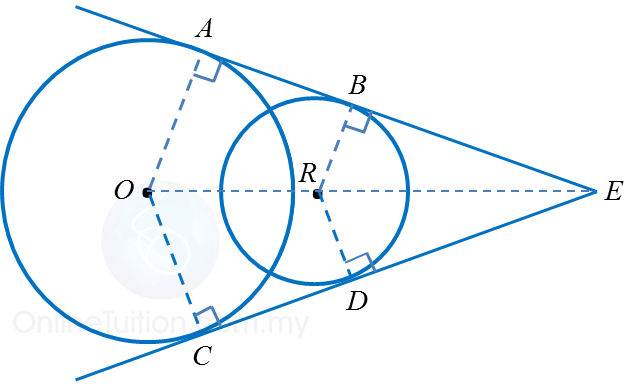# 6.5.1 Common Tangents (I)

6.5.1 Common Tangents (I)
A common tangent to two circles is a straight line that touches each of the circles at only one point.

1. Intersect at two points
(a) Circles of the same sizeNumber of common tangents Properties of common tangents Two common tangents: AB and CD AC = BD AB = CD AB parallel to // OR parallel to // CD

(b) Circles of different sizesNumber of common tangents Properties of common tangents Two common tangents: ABE and CDE AB = CD BE = DE OA // RB OC // RD

2. Intersect at one point
(a) Circles of the same sizeNumber of common tangents Properties of common tangents Three common tangents: AB, CD and PQ AC = PQ = BD AB = OR = CD AB // OR // CD AC // PQ // BD PQ perpendicular to OR

(b) Circles of different sizes
(i) External contactNumber of common tangents Properties of common tangents Three common tangents: ABE, CDE and PQ AB = CD BE = DE OA // RB OC // RD PQ perpendicular to ORE

(ii) Internal contactNumber of common tangents Properties of common tangents One common tangent: PQR ONQ perpendicular to PR